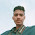# C++ Program To Find The GCD And LCM Of Two Numbers

#include <iostream.h>

int main() {
int a, b, x, y, t, gcd, lcm;

cout<<"Enter two integers\n";
cin>>x>>y;

a = x;
b = y;

while (b != 0) {
t = b;
b = a % b;
a = t;
}

gcd = a;
lcm = (x*y)/gcd;

cout<<"Greatest common divisor of "<<x<<" and "<<y<<"="<<gcd;
cout<<"Least common multiple of "<<x<<" and "<<y<<"="<<lcm;

return 0;
}

Previous Post
Next Post

#### 1 comment:

1.